# Gamma, Tonal Response Curve, and related concepts

July 4, 2021
August 9, 2022

### Introduction

In this post we discuss a number of concepts related to tonal response and gamma that are scattered around the Imatest website, making them hard to find. We’re putting them together here in anticipation of questions about gamma and related concepts, which keep arising.

Gamma (γ) is the average slope of the function that relates the logarithm of pixel levels (in an image file) to the logarithm of exposure (in the scene).

$$\text{log(pixel level)} \approx \gamma \ \text{log(exposure)}$$

This relationship is called the Tonal Response Curve, (It’s also called the OECF = Opto-Electrical Conversion Function). The average is typically taken over a range of pixel levels from light to dark gray.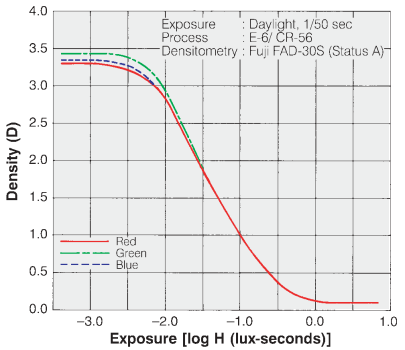Tonal Response Curve for Fujichrome Provia 100F film. The y-axis is reversed from the digital plots.

Tonal Response Curves (TRCs) have been around since the nineteenth century, when they were developed by Hurter and Driffield— a brilliant accomplishment because they lacked modern equipment. They are widely used for film. The Tonal Response Curve for Fujichrome Provia 100F film is shown on the right. Note that the y-axis is reversed from the digital plot shown below, where log(normalized pixel level) corresponds to $$D_{base} – D\text{, where } D_{base} = \text{base density} \approx 0.1$$.

Equivalently, gamma can be thought of as the exponent of the curve that relates pixel level to scene luminance.

$$\text{pixel level = (RAW pixel level)}^ \gamma \approx \text{exposure} ^ \gamma$$

There are actually two gammas: (1) encoding gamma, which relates scene luminance to image file pixel levels, and (2) display gamma, which relates image file pixel levels to display luminance. The above two equations (and most references to gamma on this page) refer to encoding gamma. The only exceptions are when display gamma is explicitly referenced, as in the Appendix on Monitor gamma.

The overall system contrast is the product of the encoding and decoding gammas. More generally, we think of gamma as contrast.

Encoding gamma is introduced in the image processing pipeline because the output of image sensors, which is linear for most standard (non-HDR) image sensors, is never gamma-encoded. Encoding gamma is typically measured from the tonal response curve, which can be obtained photographing a grayscale test chart and running Imatest’s Color/Tone module (or the legacy Stepchart and Colorcheck modules).

Display gamma is typically specified by the color space of the file. For the most common color space in Windows and the internet, sRGB, display gamma is approximately 2.2. (It actually consists of a linear segment followed by a gamma = 2.4 segment, which together approximate gamma = 2.2.) For virtually all computer systems, display gamma is set to correctly display images encoded in standard (gamma = 2.2) color spaces.

Here is an example of a tonal response curve for an in-camera JPEG for a high quality consumer camera, measured with Imatest Color/Tone.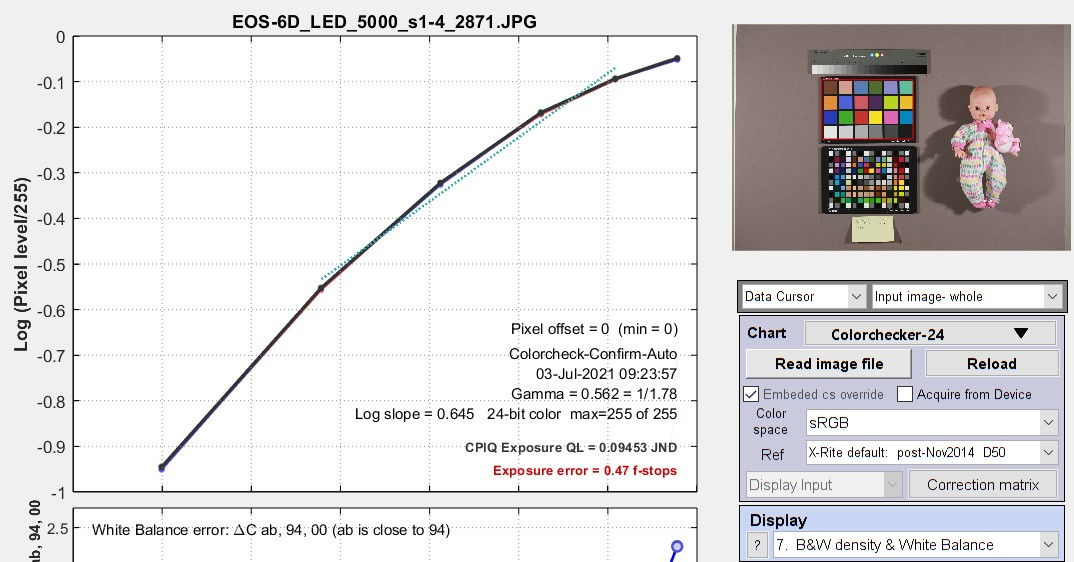Tonal response curve measured by the Imatest Color/Tone module
from the bottom row of the 24-patch Colorchecker,

Note that this curve is not a straight line. It’s slope is reduced on the right, for the brightest scene luminance. This area of reduced slope is called the “shoulder”. It improves the perceived quality of pictorial images (family snapshots, etc.) by reducing saturation or clipping (“burnout”) of highlights, thus making the response more “film-like”. A shoulder is plainly visible in the Fujichorme Provia 100D curve, above. Shoulders are almost universally applied in consumer cameras; they’re less common in medical or machine vision cameras.

Because the tonal response is not a straight line, gamma has to be derived from the average (mean) value of a portion of the tonal response curve.

### Why use logarithms?

Logarithmic curves have been used to express the relationship between illumination and response since the nineteenth century because the eye’s response to light is logarithmic. This is a result of the Weber-Fechner law, which states that the perceived change dp to a change dS in an initial stimulus S is

$$dp = dS/S$$

Applying a little math to this curve, we arrive at $$p = k \ln(S/S_0)$$  where ln is the natural logarithm (loge).

From G. Wyszecki & W. S. Stiles, “Color Science,” Wiley, 1982, pp. 567-570, the minimum light difference ΔL that can be perceived by the human eye is approximately

$$\Delta L / L = 0.01 = 1\%$$. This number may be too stringent for real scenes, where ΔL/L may be closer to 2%.

How gamma encoding increases Dynamic Range

In photography, we often talk about zones (derived from Ansel Adams’ zone system). A zone is a range of illumination L that varies by a factor of two, i.e., if the lower and upper boundaries of a zone are L1 and L2, then L2 /L1 = 2 or log2(L2) – log2(L1) = 1.

 1 2 3 4 5 6 7 8 9

Zone system chart for gamma = 2.2 (sRGB color space, etc.)

The steps between each zone has equal visual weight, except for very dark zones where the differences are hard to see.

For a set of zones z = {1, 2, 3, ..} the relative illumination of zone n is 2-(z-1) = {1, 0.5, 0.25, 0.125, …}. The illumination difference between zones is {0.5, 0.25, 0.125, …}. The scene illumination ratio is always 2:1.

 Geek alert: a lot of numbers follow— all of which are intended to show how gamma encoding increases the effective dynamic range. The total number of available pixel levels B is a function of the bit depth, B = 2(bit depth). For bit depth = 8, B = 256; for bit depth = 16, B = 65536. The relative pixel level of zone n for encoding gamma γe is bn = B × 2-(n-1)γe.For linear gamma (γe = 1), bn= {1.0, 0.5, 0.25, 0.125, …} (the same as L). For γe= 1/2.2 = 0.4545, bn = {1.0000, 0.7297, 0.5325, 0.3886, 0.2836, 0.2069, 0.1510 ,0.1102, …}. The relative pixel levels bn decrease much more slowly than for γe = 1. The relative number of pixel levels in each zone is npγ(i) = bn(i) – bn(i+1), which brings us to the heart of the issue. For a maximum  pixel level of 2(bit depth)-1 = 255 for widely-used files with bit depth = 8 (24-bit color), the total number of pixels in each zone is Npγ(i) = 2(bit depth)npγ(i).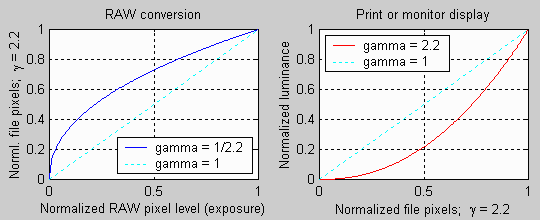When gamma = 2.2, the darker zones in the file contain more pixel levels. For linear images (γe = 1), npγ(i) = {0.5, 0.25, 0.125, 0.0625, …}, i.e., half the pixel levels would be in the first zone, a quarter would be in the second zone, etc. For files with bit depth = 8, the zones starting from the brightest would have Npγ(i) = {128, 64, 32, 16, 8, 4, 2, 1 …} pixel levels of 256 (0-255). By the time you reached the 5th or 6th zone, the spacing between pixel levels would be small enough to cause significant “banding”, limiting the dynamic range. For images encoded with γe = 1/2.2 = 0.4545, the relative number of pixels in each zone would be bn(i) = {0.2703, 0.1972, 0.1439, 0.1050, 0.0766, 0.0559, 0.0408, 0.0298, 0.0217, 0.0159…}, and the total number Npγ(i) would be {69.2, 50.5, 36.8, 26.9, 19.6, 14.3, 10.4, 7.63, 5.56, 4.06, …} of 256. The sixth zone, which has only 4 levels for γe = 1, has 14.3 levels. The 10th gamma-encoded zone has the same number of levels (4) as the 6th linear zone, i.e., an effective dynamic range increase of up to four zones (f-stops; factors of 2). To summarize:

 These numbers show how gamma-encoding greatly improves the effective dynamic range of images with limited bit depth by redistributing pixel levels so there are more in darker zones  and fewer in lighter zones (which have way more than needed in linear systems).

Of course, the improvement will be less if flare light in dark regions is the limiting factor.

### What gamma (and Tonal Response Curve) should I expect? And what is good?

JPEG images from consumer cameras typically have complex tonal response curves (with shoulders), with gamma (average slope) in the range of 0.45 to 0.6. This varies considerably for different manufacturers and models. The shoulder on the tonal response curve allows the the slope in the middle tones to be increased without worsening highlight saturation. This increases the apparent visual contrast, resulting in “snappier” more pleasing images.

RAW images from consumer cameras have to be decoded using LibRaw/dcraw. Their gamma depends on the Output gamma and Output color space settings (display gamma is shown in the settings window). Typical results are

• Encoding gamma ≅ 0.4545 with a straight line TRC (no shoulder) if conversion to a color space (usually sRGB or Adobe RGB) is selected;
• Encoding gamma ≅ 1.0 if a minimally processed image is selected.

RAW images from binary files (usually from development systems) have straight line gamma of 1.0, unless the Read Raw Gamma setting (which defaults to 1) is set to a different value.

Flare light can reduce measured gamma by fogging shadows, flattening the Tonal Response Curve for dark regions. Care should be taken to minimize flare light when measuring gamma.

That said, we often see values of gamma that differ significantly from the expected values of ≅0.45-0.6 (for color space images) or 1.0 (for raw images without gamma-encoding). It’s difficult to know why without a hands-on examination of the system. Perhaps the images are intended for special proprietary purposes (for example, for making low contrast documents more legible by increasing gamma); perhaps there is a software bug.

### Gamma and MTF measurement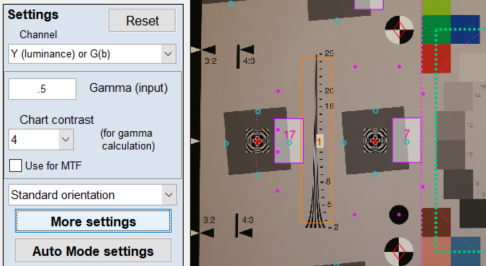MTF (Modulation Transfer Function, which is equivalent to Spatial Frequency Response), which is used to quantify image sharpness, is calculated assuming that the signal is linear. For this reason, gamma-encoded files must be linearized, i.e., the gamma encoding must be removed. The linearization doesn’t have to be perfect, i.e., it doesn’t have be the exact inverse of the tonal response curve. For most images (especially where the chart contrast is not too high), a reasonable estimate of gamma is sufficient for stable, reliable MTF measurements. The settings window for most MTF calculation has a box for entering gamma (or setting gamma to be calculated from the chart contrast).

Gamma is entered in the Setup or More settings window for each MTF module. They are described in the documentation for individual Rescharts modules. For Slanted-edge modules, they appear in the Setup window (crop shown on the right) and in the More settings window (crop shown below).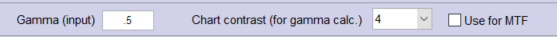Gamma (input) defaults to 0.5, which is a reasonable approximation for color space files (sRGB, etc.), but is incorrect for raw files, where gamma ≅ 1. Where possible we recommend entering a measurement-based estimate of gamma.

#### Determine gamma from a grayscale pattern

Gamma is often measured from grayscale patterns, which can be in separate charts or included in any of several sharpness charts— SFRplus, eSFR ISO, Star, Log F-Contrast, and Random (but not Checkerboard). The grayscale pattern from the eSFR ISO and SFRplus chart is particularly interesting because it shows the levels of the light and dark portions of the slanted-edge patters used to measure MTF.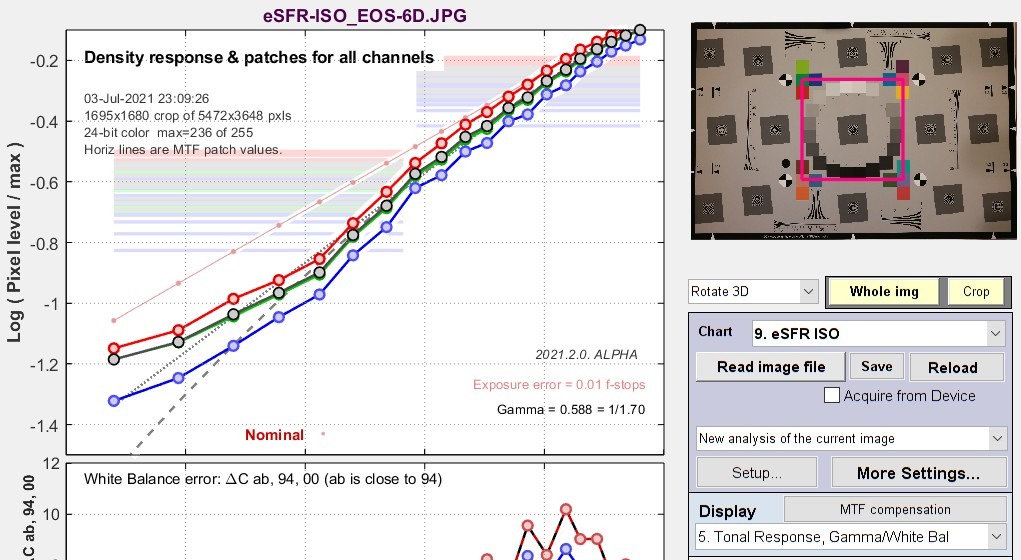Tonal response plot from eSFR ISO chart

Gamma = 0.588 here: close to the value (shown above) measured from the X-Rite Colorchecker for the same camera. The interesting thing about this plot is the pale horizontal bars, which represent the pixel levels of the light and dark portions of the selected slanted-edge ROIs (Regions of Interest). This lines let you see if the slanted-edge regions are saturating or clipping. This image shows that there will be no issue.

#### Determine gamma from each individual slanted-edge

Enter chart contrast and check Use for MTF. Only available for slanted-edge MTF modules. When Use for MTF is checked, the gamma (input) box is disabled.

This setting uses the measured of contrast of flat areas P1 and P2 (light and dark portions of each individual slanted-edge Region of Interest (ROI), away from the edge itself) to calculate the gamma for each edge. It is easy to use and quite robust. The only requirement is that the printed chart contrast ratio be known and entered. (It is 4:1 or 10:1 for nearly all Imatest slanted-edge charts.) This method is not reliable for chart contrasts higher than 10:1.

$$\displaystyle gamma\_encoding = \frac{\log(P_1/P_2)}{\log(\text{chart contrast ratio)}}$$

 A brief history of chart contrast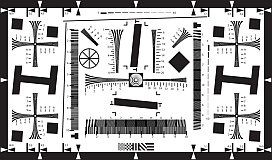The ISO 12233:2000 standard called for a chart contrast of at least 50:1. This turned out to be a poor choice: The high contrast made it difficult to avoid clipping (flattening of the tonal response curve for either the lightest or darkest areas), which exaggerates MTF measurements (making them look better than reality). There is no way to calculate gamma from the ISO 12233:2000 chart (shown on the right). This issue was finally corrected with ISO 12233:2014 (later revisions are relatively minor), which specifies a 4:1 edge contrast, which not only reduces the likelihood of clipping, but also makes the MTF calculation less sensitive to the value of gamma used for linearization. The old ISO 12233:2000 chart is still widely used: we don’t recommend it.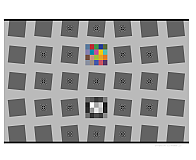The SFRplus chart, which was introduced in 2009, originally had an edge contrast of 10:1 (often with a small number of 2:1 edges). After 2014 the standard contrast was changed to 4:1 (shown on the right). The eSFR ISO chart (derived from the 2014 standard) always has 4:1 edge contrast. Both SFRplus and eSFR ISO have grayscales for calculating tonal response and gamma. SFRreg and Checkerboard charts are available in 10:1 and 4:1 contrast. Advantages of the new charts are detailed here and here. Imatest chrome-on-glass (CoG) charts have a 10:1 contrast ratio: the lowest that can be manufactured with CoG technology. Most other CoG charts have very high contrast (1000:1, and not well-controlled). We don’t recommend them. We can produce custom CoG charts quickly if needed.

### Which patches are used to calculate gamma?

This is an important question because gamma is not a “hard” measurement. Unless the Tonal Response Curve says pretty close to a straight line log pixel level vs. log exposure curve, its measured value depends on which measurement patches are chosen. Also, there have been infrequent minor adjustments to the patches used for Imatest gamma calculations— enough so customers occasionally ask about tiny discrepancies they find.

For the 24-patch Colorchecker, patches 2-5 in the bottom row are used (patches 20-23 in the chart as a whole).

For all other charts analyzed by Color/Tone or Stepchart, the luminance Yi for each patch i (typically 0.2125×R + 0.7154×G + 0.0721×B) is found, and the minimum value Ymin, maximum value Ymax, and range Yrange = YmaxYmin is calculated. Gamma is calculated from patches where Ymin + 0.2×Yrange  < Yi  < Ymax – 0.1×Yrange . This ensures that light through dark gray patches are included and that saturated patches are excluded.

### History: where did gamma ≅ 2.2 come from?

It came from the Cathode Ray Tubes (CRTs) that were universally used for television and video display before modern flat screens became universal. In CRTs, screen brightness was proportional to the control grid voltage raised to the 2 to 2.5 power. For this reason, signals had to be encoded with the approximate inverse of this value, and this encoding stuck. As we describe above, in “What is gained by applying a gamma curve?”, there is a real advantage to gamma encoding in image files with a limited bit depth, especially 8-bit files, which only have 256 possible pixel levels (0-255).

### Why are images converted from raw files often darker then JPEGs from the same capture?

Many cameras have the option of producing both interchangeable image files (almost always JPEG) and raw files from the same image capture. The raw-converted files are frequently darker— sometimes much darker— than the corresponding JPEGs. This is not an accident.

In Imatest, raw files are typically converted to interchangeable files (usually TIFF) using LibRaw, which has several presets and additional options. The most commonly-used presets are Color 24-bit sRGB (gamma ≅ 2.2) and Color 48-bit Adobe RGB (gamma = 2.2). These settings use EXIF metadata in the raw file to perform white balance and color correction (for the selected color space) and apply an appropriate straight-line gamma curve, $$\text{log(pixel level)} = \gamma \ \text{log(exposure)}$$ . The tonal response curve, displayed logarithmically, is a straight line with no “shoulder” or other curvature.

JPEGs, on the other hand, frequently have a “shoulder”— a region where gamma (contrast) is gradually reduced— in the highlights. The shoulder improves the perceived pictorial quality of the image by reducing saturation (or “burnout”) of the highlights. It was a part of film response curves, long taken for granted. In order to achieve this shoulder, the exposure has to be reduced below the optimum level for a straight gamma curve to allow some “headroom” for the shoulder (before highlights are saturated). Here is an example:  in-camera JPEG and LibRaw-converted raw images of the Colorchecker charts and doll, whose tonal response curve are shown above or the in-camera JPEG and below for the LibRaw-converted TIFF.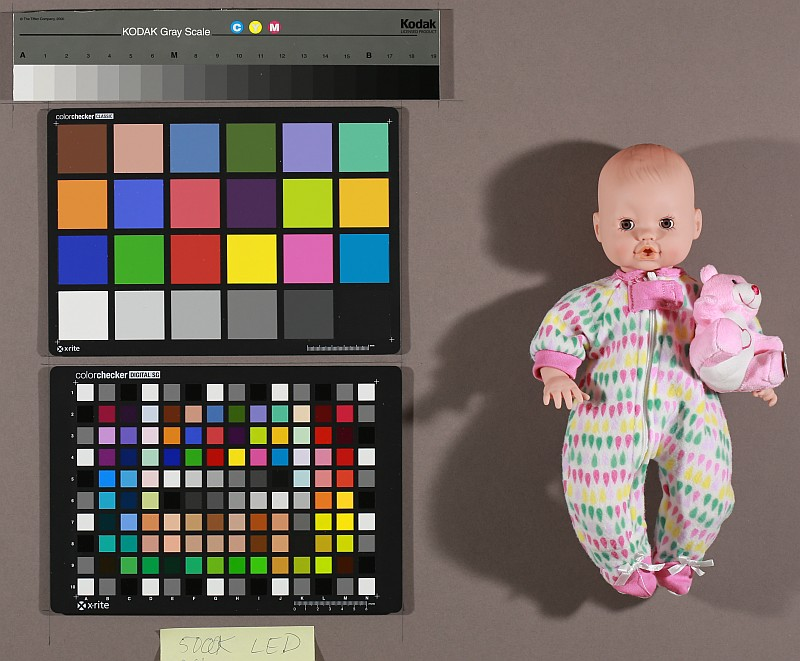in-camera JPEG. Tonal response curveabove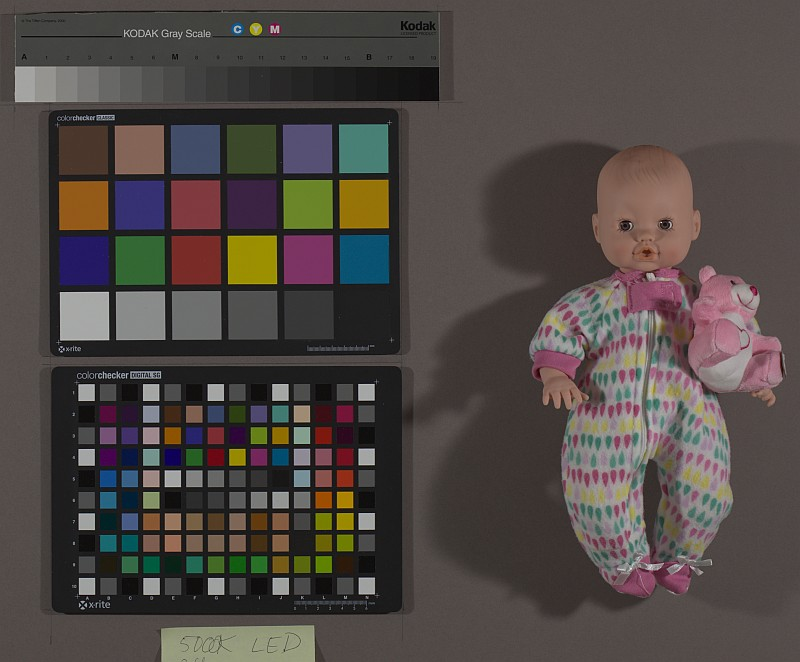converted from CR2 raw with LibRaw. Tonal response below

Here is the (nearly) straight-line density response for the converted CR2 raw image on the right. Though this image is typical, we have seen much larger differences between JPEG and converted raw files.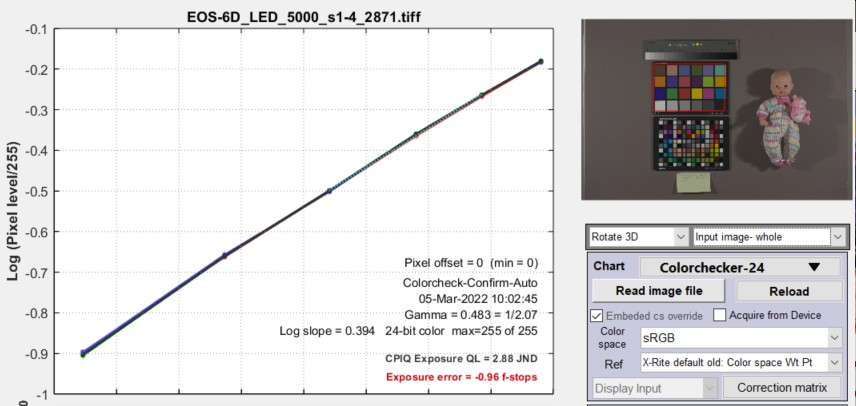Tonal response curve from the bottom row of the Colorchecker
from the image on the right, above (converted from raw with LibRaw)

### Tone mapping

Tone mapping  is a form of nonuniform image processing that lightens large dark areas of images to make features more visible. It reduces global contrast (measured over large areas) while maintaining local contrast (measured over small areas) so that High Dynamic Range (HDR) images can be rendered on displays with limited dynamic range. it can usually be recognized by extremely low values of gamma (<0.25; well under the typical values around 0.45 for color space images), measured with standard grayscale charts.

Tone mapping can seriously spoil gamma and Dynamic Range measurements, especially with standard grayscale charts. The Contrast Resolution chart was designed to give good results (for the visibility of small objects) in the presence of tone mapping, which is becoming increasingly popular with HDR images.

### Logarithmic encoding

Logarithmic encoding, which has a similar intent to gamma encoding, are widely used in cinema cameras. According to Wikipedia’s Log Profile page, every camera manufacturer has its own flavor of logarithmic color space. Since they are rarely, if ever, used for still cameras, Imatest does little with them apart from calculating the log slope (n1, below). A detailed encoding equation from renderstory.com/log-color-in-depth is $$f(x) = n_1 \log(r_1 n_3+ 1) + n_2$$.

From context, this can be expressed as

$$\text{pixel level} = p = n_1 \log(\text{exposure} \times n_3+ 1) + n_2$$

Since log(0) = -∞, exposure must be greater than 1/n3 for this equation to be valid, i.e., there is a minimum exposure value (that defines a maximum dynamic range).

By comparison, the comparable (simplified) equation for gamma encoding is

$$\log(\text{pixel level}) = \log(p) = \gamma \log(\text{exposure}) \ ; \ \ \ \ \ \ p = \text{exposure}^{\gamma}$$

The primary difference is that pixel level instead of log(pixel level) is used the equation. The only thing Imatest currently does with logarithmic encoding is to display the log slope n1, shown in some tonal response plots. Imatest could do more on customer request.

Logarithmic encoding has a very different appearance from gamma encoding when viewed in log-log density plots, as shown on the right. These curves are useful for identifying logarithmic encoding. The plot below is a density response curve, made with an Imatest dynamic range chart, for a camera that produces still and video output. At this time we’re not sure it’s intentionally logarithmic, but it certainly looks it. The flattened response and steps on the left are caused by flare light.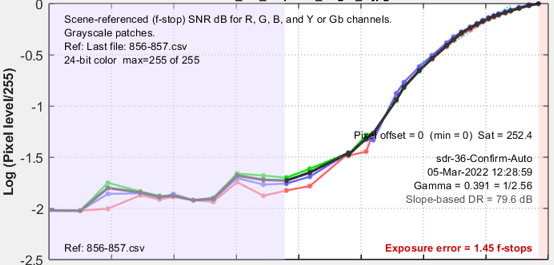Response of an MP4 frame from a camera that produces both still and video images.

Dynamic range limitation  An issue with logarithmic spaces is that their dynamic range is strictly limited by the math since log(0) = -∞. Using the above equation and assuming that log represents log10 and n2 = 1

$$p = \gamma \ \log_{10}(exp)+1$$

If we assume that exp is normalized to a maximum value of expmax = 1,  p(expmax) = p(1) = 1. The darkest level that can be reproduced is p(expmin) = γ log10(expmin)+1 = 0.

$$\displaystyle \log_{10}(exp_{min}) = -1/\gamma \ ; \ \ \ \ exp_{min} = 10^{-1/\gamma}$$

For gamma = 0.5 (shown in the plot, above right), expmin = 10-2 = 0.01, equivalent to

$$\text{Dynamic Range} = exp_{max}/exp_{min} = 1/0.01 = 100 = 40\text{dB}$$

#### Logarithmic response example

interceptor121.com/2021/02/07/the-truth-about-v-log/ has detailed equations for Panasonic V-LOG encoding. We programmed them because

1. it’s a real example and
2. it was easy.

The curve is a hybrid curve with a logarithmic section for illumination above cut1 = 0.01 and a linear section below cut1. In this respect it is somewhat similar to sRGB, which has linear and gamma sections that average to approximately gamma = 2.2.

The encoding is intended to improve dynamic range, but we have some doubts because the slope of the log-log plot is very low for the darkest regions (x < 10-3).

 This brings us to the big question: Why is logarithmic encoding widely used in video/cinema systems? Coming from still photography, where gamma encoding is widely used (but log encoding isn’t), we are aware that gamma encoding accomplishes the same goals without the dynamic range limitation. If you have a good answer, please

 Misinformation warning — The Internet is full of misinformation about logarithmic color spaces. A good example is the B&H page, Understanding Log-Format Recording, which says, ” If your video—like most video—is not recorded using a log picture profile, chances are the exposure is being recorded in a linear fashion.” This is completely incorrect.  The author seems to have no inkling that gamma encoding exists. Then he compounds the confusion with statements like “The problem arises with the realization that the exposure values with which we measure light are not linear. An exposure “stop” represents a doubling or halving of the actual light level, not an increase by some arbitrary linear value on a scale. You could, in fact, say exposure values are themselves logarithmic!”.  Exposure Value (EV) is a relative measurement unit based on factors 2 (log2(exposure level)) that corresponds to the sensitivity of the human eye. It has nothing to do with the camera itself.  For the record: Most digital sensors have a linear response, with more than 8 bits for high quality cameras (12, 14, or more bits are common). Following Analog-to-Digital conversion, most still cameras encode the image using a gamma curve. Video cameras use either gamma or log curves. As we mentioned above, this extends the effective dynamic range for gamma encoding.

### Appendix I: Monitor gamma

Monitors are not linear devices. They are designed so that Brightness is proportional to pixel level raised to the gamma power, i.e., $$\text{Brightness = (Pixel level)}^{\gamma\_display}$$.

For most monitors, gamma should be close to 2.2, which is the display gamma of the most common color spaces, sRGB (the Windows and internet standard) and Adobe RGB.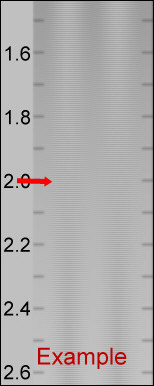The chart on the right is designed for a visual measurement of display gamma. But it  rarely displays correctly in web browsers. It has to be displayed in the monitor’s native resolution, 1 monitor pixel to 1 image pixel. Unfortunately, operating system scaling settings and browser magnifications can make it difficult. To view the gamma chart correctly, right-click on it, copy it, then paste it into Fast Stone Image Viewer. This worked well for me on my fussy system where the main laptop monitor and my ASUS monitor (used for these tests) have different scale factors (125% and 100%, respectively). The gamma will be the value on the scale where the gray area of the chart has an even visual density. For the (blurred) example on the left, gamma = 2. Although a full monitor calibration (which requires a spectrophotometer) is recommended for serious imaging work, good results can be obtained by adjusting the monitor gamma to the correct value. We won’t discuss the process in detail, except to note that we have had good luck with Windows systems using QuickGamma.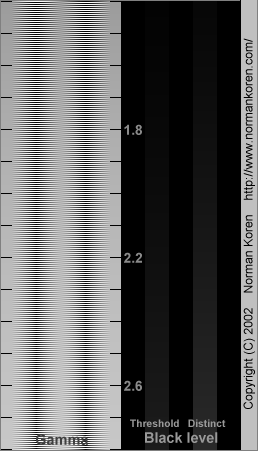Gamma chart. Best viewed with Fast Stone image viewer.

#### Appendix II: Tonal response, gamma, and related quantities

For completeness, we’ve updated and kept this table from elsewhere on the (challenging to navigate) Imatest website.

Parameter Definition
Tonal response curve The pixel response of a camera as a function of exposure. Usually expressed graphically as log pixel level vs. log exposure.
Gamma

Gamma is the average slope of log pixel levels as a function of log exposure for light through dark gray tones). For MTF calculations It is used to linearize the input data, i.e., to remove the gamma encoding applied by image processing so that MTF can be correctly calculated (using a Fourier transformation for slanted-edges, which requires a linear signal).

Gamma defaults to 0.5 = 1/2, which is typical of digital camera color spaces, but may be affected by image processing (including camera or RAW converter settings) and by flare light. Small errors in gamma have little effect on MTF measurements (a 10% error in gamma results in a 2.5% error in MTF50 for a normal contrast target). Gamma should be set to 0.45 or 0.5 when dcraw or LibRaw is used to convert RAW images into sRGB or a gamma=2.2 (Adobe RGB) color space. It is typically around 1 for converted raw images that haven’t had a gamma curve applied. If gamma  is set to less than 0.3 or greater than 0.8, the background will be changed to pink to indicate an unusual (possibly erroneous) selection.

If the chart contrast is known and is ≤10:1 (medium or low contrast), you can enter the contrast in the Chart contrast (for gamma calc.) box, then check the Use for MTF (√) checkbox. Gamma will be calculated from the chart and displayed in the Edge/MTF plot.

If chart contrast is not known you should measure gamma from a grayscale stepchart image. A grayscale is included in SFRplus, eSFR ISO and SFRreg Center ([ct]) charts. Gamma is calculated and displayed in the Tonal Response, Gamma/White Bal plot for these modules. Gamma can also be calculated from any grayscale stepchart by running Color/Tone Interactive, Color/Tone Auto, Colorcheck, or Stepchart. [A nominal value of gamma should be entered, even if the value of gamma derived from the chart (described above) is used to calculate MTF.]

 Gamma Gamma is the exponent of the equation that relates image file pixel level to luminance. For a monitor or print, $$\displaystyle \text{Output luminance = (pixel level)}^{gamma\_display}$$ When the raw output of the image sensor, which is typically linear, is converted to image file pixels for a standard color space, the approximate inverse of the above operation is applied. $$\displaystyle \text{Pixel level = (RAW pixel level)}^{gamma\_encoding} \approx exposure\ ^{gamma\_encoding}$$ This is equation is an approximation because the tonal response curve (which often has a “shoulder”— a region of decreased contrast in the highlights) doesn’t follow the gamma equation exactly. It is often a good approximation for light to dark gray tones: good enough for to reliably linearize the chart image if the edge contrast isn’t too high (4:1 is recommended in the ISO 12233:2014+ standard). $$\text{Total system contrast} = gamma\_encoding \times gamma\_display$$. The most common value of of display gamma is 2.2 for color spaces used in Windows and the internet, such as sRGB (the default) and Adobe RGB (1998). In practice, gamma is equivalent to contrast.  When the Use for MTF checkbox (to the right of the Chart contrast dropdown menu) is checked, camera gamma is estimated from the ratio of the light and dark pixel levels P1 and P2 in the slanted-edge region (away from the edge) if the chart contrast ratio (light/dark reflectivity) has been entered (and is 10:1 or less). Starting with $$P_1/P_2 = \text{(chart contrast ratio)}^{gamma\_encoding}$$, $$\displaystyle gamma\_encoding = \frac{\log(P_1/P_2)}{\log(\text{chart contrast ratio)}}$$

Shoulder A region of the tonal response near the highlights where the slope may roll off (be reduced) in order to avoid saturating (“bunring out”) highlights. Frequently found in pictorial images. Less common in machine vision images (medical, etc.) When a strong shoulder is present, the meaning of gamma is not clear.

This site uses Akismet to reduce spam. Learn how your comment data is processed.